RUSSIAN JOURNAL OF EARTH SCIENCES, VOL. 19, ES6006, doi:10.2205/2019ES000683, 2019

# On different approaches to statistical description of ocean waves

Andrei Pushkarev1,2

1Skoltech, Moscow, Russia

2Lebedev Physical Institute RAS, Moscow, Russia

### Abstract

We analyze well-known model for wind energy input and wave-breaking absorption in energy transfer equation via its numerical comparison with recently developed alternative model. The comparison is done for time and space-independent velocity of the wind for the waves growing along the fetch coordinate. Significant differences have been found for integral as well as spectral characteristics of these models. It is shown that slight modification of the analyzed model significantly improves its properties and provides better description of the physical situation.

### Introduction

The process of energy transfer between deep gravity ocean surface waves spectral components, in the absence of the ocean currents, is described by the energy transfer equation, or Hasselmann Equation, hereafter HE, [Hasselmann, 1962]:

\begin{equation} \tag*{(1)} \frac{\partial \varepsilon }{\partial t} +{\vec{C_g}} \nabla_{\vec{r}} \varepsilon = S_{total} \end{equation}

where $\varepsilon =\varepsilon (\vec{k},\vec{r},t)$ is the wave energy spectral density, depending on 2D vectors $\vec{r}=(x,y)$ and $\vec{k}=(k_x,k_y)$, in real and Fourier spaces, correspondingly, and time $t$. $S_{total}$ is the total source term

\begin{equation} \tag*{(2)} S_{total} = S_{nl} + S_{ds} + S_{in} \end{equation}

where $S_{nl}$ is nonlinear four-waves interaction term, $S_{ds}$ is the wave-breaking wave energy absorption term, and $S_{in}$ is the wind energy input term.

For the last 30 years, Eq.(1) is the hull of the operational ocean wave prediction models [Tolman, 2013; SWAN, 2015] though, there is no general agreement on the form of $S_{ds}$ and $S_{in}$ terms.

It was recently shown that $S_{nl}$ is the dominant term in Eq.(1) [Zakharov, 2010; Zakharov and Badulin, 2011]. Therefore, the first approximation of Eq.(1) is $S_{nl}=0$, it plays important role in the theory of weak turbulence. It's basic solution is the spectrum [Zakharov and Filonenko, 1967]:

\begin{eqnarray*} \varepsilon \simeq \frac{P^{1/3}}{\omega^4} \end{eqnarray*}

where $P$ is the energy flux to high frequencies. Since then, its angular-dependent generalizations have been developed [Kats and Kontorovich, 1974; Kats et al., 1975].

The knowledge of the exact expression for nonlinear interaction $S_{nl}$ is of little help for operational models due to its computational overhead. Instead, simpler substitutes of $S_{nl}$, such as DIA and the likes, are used for real-time operational prediction. As the results, there is the need for tuning coefficient in front of them. However, several researchers reported their failure to reproduce the original $S_{nl}$ properties.

There is another negative side of such $S_{nl}$ deformation with even more severe consequences: due to distortions of $S_{nl}$ as the leading term, the other source terms $S_{in}$ and $S_{diss}$ need to be distorted in a way to compensate $S_{nl}$ deformation to reach decent approximation of Eq.(1) in specific situation. Such manipulations lead to the loss of physical properties and universality of the original model.

Therefore, while $S_{nl}$ is rigorously analytically formulated, but not used, the wind input $S_{in}$ and wave-breaking absorption term $S_{ds}$ are poorly formulated, incorporate heuristic assumptions, and include many tunable parameters. The development of good $S_{in}$ theory was complicated by understudied sea surface boundary layer, uncorrelated with surface waves. The examples of the development of various $S_{in}$ terms contain plenty of heuristic suggestions about the amplitude and angular distribution of waves. As the consequence, the local values of different parameterizations of $S_{in}$ vary up to the factor of 5 [Badulin et al., 2005; Pushkarev and Zakharov, 2016]. The discussion and demonstration of these facts can be found in [Gagnaire-Renou et al., 2011; Pushkarev and Zakharov, 2016].

As with $S_{in}$, there is no agreement about parametrization of $S_{ds}$. While the major wave energy absorption mechanism, the scientists agree on, is the wave breaking, one can find in the literature another ambiguous heuristic long-waves absorption mechanism as well [Tolman and Chalikov, 1996]. Moreover, there is no consent at the moment on the localization of the wave-breakings in wave-number space. As far as concerns operational models, the dominant part of the absorption happens in the vicinity of the spectral peak. This fact contradicts, however, the reports that such assumption does not satisfy the nonlinear tests of the Eq.(1) [Pushkarev and Zakharov, 2016; Dyachenko et al., 2015].

Due to the above mentioned facts, the operational models obey dozens of tuning parameters. Therefore, there is the emerging feeling that the new generation of statistical justified approaches to waves modeling should be developed.

Recently, the new ZRP approach to the formulation of $S_{in}$ and $S_{ds}$ balanced terms has been offered by Zakharov et al. . It does not use turbulent layer analytical theory, or precise measurement of the boundary layer. Instead, it is based on the existence of two-parameter automodel solutions of the Eq.(1) and their restriction to the one-parameter automodel solution through experimental data. This solution can be written as:

\begin{eqnarray} \tag*{(3)} \epsilon = \chi^{p+q} F(\omega \chi^q) \end{eqnarray} \begin{eqnarray} \tag*{(4)} 10q-2p = 1, \,\,\,\, q=\frac{1}{2+s} \end{eqnarray} \begin{eqnarray*} p = 1, \,\,\, q = 3/10, \,\,\,\,s=4/3 \end{eqnarray*} \begin{eqnarray*} E(\chi) = E_0 \chi^p \end{eqnarray*} \begin{eqnarray} \tag*{(5)} <\omega(\chi)> = \omega_0 \chi^{-q} \end{eqnarray}

where $E(\chi)$ and $<\omega(\chi)>$ are the full wave energy of waves and the average frequency, as the dimensionless coordinate $\chi = xg/U^2$ functions, where $x$ is the regular dimensional coordinate in meters of the fetch, $U$ is the wind velocity and $g$ is gravitational acceleration; $s=4/3$ is the power of exponent for $S_{in}=\omega^{s+1}$ dependence on frequency $\omega$; $E_0$ and $\omega_0$ are the constant coefficients.

Later, we will compare ZRP approach [Zakharov et al., 2017] with M1 and M2 approaches. M1 approach uses $S_{in}$ and $S_{ds}$ based on [Donelan et al., 2012]. M2 approach is the "artificial" one, using the properties of ZRP and M1 approaches.

Let us note that ZRP and M1 approaches use different physics: ZRP model presumes the dominance of the nonlinear interactions and the flux of the energy from the spectral peak area toward the absorption area of big wave numbers, whereas M1, as shows the numerical simulation, absorbs the vast portion of the wave energy in the area of the intermediate wave numbers, nearer the area of the spectral peak.

That leads to substantial discrepancy in full wave energy and average frequency dependencies on the coordinate of the fetch, as well as the difference in the wave energy spectra.

The simulations of the "artificial" M2 approach exhibits the improvements of the properties of M1 approach toward better automodel behavior as well as wave energy spectrum properties, and advice the usage of high wave-numbers wave energy absorption mechanism in Eq.(1).

Below, we explain the above mentioned approaches and present the supportive evidence for our vision of the studied subject.

### The models formulation and numerical approach

The numerical model used the stationary case of Eq.(1):

\begin{eqnarray} \tag*{(6)} \frac{1}{2} \frac{\omega }{k} \cos \theta \frac{\partial \varepsilon}{\partial x} = S_{nl} + S_{wind} + S_{diss} \end{eqnarray}

It is well-known that numerical solution of Eq.(6) is complicated by the presence of the singularity in the form of $\cos \theta$, turning to $0$ in the case of $\theta=\pm\pi/2$, which was historically resolved [Banner and Young, 1975] via limitation of the Fourier space domain to the angular spread of $-60^\circ < \theta < 60^\circ$, and zeroing out the remaining part. Such approach allows to avoid the division of the right hand side of Eq.(6) by $0$ at $\pm\pi/2$. Due to the assumption of major amount of waves propagating in the wind direction, this approach looks sensible. In our simulation, we used the angular spread of $-90^\circ < \theta < 90^\circ$.

WRT (Webb-Resio-Tracy) method [Tracy and Resio, 1982] has been applied for the calcualation of $S_{nl}$ part in its original exact formulation. It used $71$ logarithmically placed frequency points in the range $0.1\,\,\,Hz < f < 2.0\,\,\,Hz$ and $36$ uniformly positioned angular points in the range $0 < \theta < 2\pi$. We used time-independent spatial step of $1$ m or $2$ meters to advance explicitly in real space with the accuracy of first order.

Initial conditions were chosen in the form of homogeneous low-level white noise distribution of energy in Fourier space $\varepsilon(\omega,\theta)=10^{-6}\;\;m^4$. The permanent wind with the velocity of $10 \,\, \rm m/sec$ was supposed to be blowing away from the coast line.

#### ZRP model

For the wind input term $S_{in}$, the recently developed through automodel approach, ZRP wind input term has been utilized [Zakharov et al., 2017; Pushkarev and Zakharov, 2016]:

\begin{equation} \tag*{(7)} S_{in}^{ZRP}(\omega,\theta) = \gamma(\omega,\theta)\cdot \varepsilon(\omega,\theta) \end{equation}

\begin{equation} \tag*{(8)} \gamma(\omega,\theta ) = \left\{ \begin{array}{l} \displaystyle{0.05 \frac{\rho_a}{\rho _w} \omega \left(\frac{\omega }{\omega _{0} } \right)^{4/3} q(\theta ) {\rm \; \; for}} f_{min} \leqslant f \leqslant f_{d}, \; \; \omega = 2 \pi f {0{\rm \; \; otherwise}} \end{array}\right. \end{equation}

\begin{equation} \tag*{(9)} q(\theta ) = \left\{\begin{array}{l} {\cos 2\theta {\rm \; \; for\; -}\pi {\rm /4}\leqslant \theta \leqslant \pi {\rm /4}} {0{\rm \; \; otherwise}} \end{array}\right. \end{equation} \begin{eqnarray*} &&\omega _{0} = \frac{g}{U }, \,\,\, \frac{\rho_a}{\rho_w} =1.3\cdot 10^{-3} \end{eqnarray*}

$U=10$ m/sec is the velocity of wind at $10$ meters height above the water surface, $\rho_a$ and $\rho_w$ are the densities of air and water, $f_{min}=0.1$ Hz and $f_d=1.1$ Hz.

As far as concerns the wave-breaking absorption function in ZRP model, it was used in the "implicit" way. It was simulated via extension of the wave energy spectrum from $\omega_{d}$ by the law $A(\omega_{d}) \cdot \omega^{-5}$, which is known as Phillips law [Phillips, 1966]. Due to the fact that this function decays more rapidly than equilibrium law $\omega^{-4}$, it provides short-wave energy absorption, for wave energy cascade, arriving to that region due to nonlinear four-waves interactions.

There is no need to know the value of the coefficient $A(\omega_{d})$ before $\omega ^{-5}$ in the explicit form. It is automatically dynamically determined through the requirement of the wave energy spectrum to be continuous at $\omega_{d}$ on every discrete time step. Or, saying the same things the other way, the Phillips spectrum beginning point has to be the same as the ending frequency point $f_{d} \simeq 1.1\,\,Hz$ of the spectrum, which is subject to change at every time step.

#### M1 approach

The well-known M1 model [Donelan et al., 2012] will be concisely outlined in this section for reader convenience.

The wind input source is defined by

\begin{equation} \tag*{(10)} S_{in}^{M1} = A_1 (U_{\lambda/2} \cos \theta - c ) |U_{\lambda/2} \cos \theta - c | \frac{k \omega}{g} \frac{\rho_a}{\rho_w} \varepsilon(k, \theta) \end{equation}

where $\theta$ is the angle between wind direction and wave vector $k$, $A_1=0.11$ is known as the sheltering coefficient, $U_{\lambda/2}$ is the wind velocity at one half of wavelength above the surface, assuming the logarithmic profile

\begin{eqnarray*} U_{\lambda/2} = \frac{U_*}{\kappa} \ln \frac{z}{z_0} \nonumber \end{eqnarray*}

where $U_{*}$ is the friction velocity, $\kappa=0.41$ is Von Karman constant, $z= \frac{1}{2} \lambda$ is the elevation equal to a fixed fraction $\frac{1}{2}$ of the spectral peak wavelength $\lambda=2\pi/k_p$, where $k_p$ is the spectral peak wave-number. $z_0=\alpha_C u_{*}^2/g$ is the surface roughness, where Charnock constant $\alpha_C = 0.015$ [Charnock, 1955].

The absorption is described by "spilling breakers" function [Donelan et al., 2012]

\begin{eqnarray*} S_{sb}^{M1} = -A_2 \left [ 1+ A_3 {MSM}^2(k,\theta) \right ]^2 \times \end{eqnarray*}

\begin{equation} \tag*{(11)} \times \left[B(k,\theta)\right]^{2.53} \omega(k) \varepsilon(k, \theta) \end{equation}

where

\begin{eqnarray*} {MSM}^2(k,\theta) = \int_0^{k} p^2 \varepsilon(p,\theta) dp \end{eqnarray*}

is the Mean Square Slope (MSS) in the direction $\theta$ of all waves longer than $\displaystyle{\frac{2 \pi}{k}}$, $B(k,\theta) = k^4 \varepsilon(k,\theta)$ is the degree of saturation, $A_2=46.665$, $A_3 = 240$.

#### Difficulties of M1 approach numerical simulation

Analytical formulation of M1 approach itself, without getting into physical details of its justification, is difficult for numerical simulation in the straighforward manner, when the wave energy dissipation Eq.(11) is considered as the part of the "whole" $S_{tot}$ source function Eq.(2).

The reason is connected with the "degree of saturation" $B(k,\theta) = k^4 \varepsilon(k,\theta)$, which was constructed in the assumption of fast enough decay of the spectrum $\varepsilon(k,\theta)$ as the function of wave number $k$. Numerical simulation of waves turbulence excitation, which starts from the low level of "seeding waves", exites initial wave spectrum in high frequencies domain, where there is no fast decaying spectrum yet – it appears at later stages of the spectral evolution, following the spectral maximum down-shift.

Therefore, due to the presence of fast growing factor $\omega^{21.24}$ in Eq.(11), the numerical simulation of M1 model exhibits "blow-up" instabilities right on its start from low-level "seeding waves" conditions.

In this relation, another interpretation of the nonlinear absorption function Eq.(11) happenes to be effective in this case: it is possible to solve analytically the absorptional part of Eq.(1)

\begin{eqnarray*} \frac{1}{2} \frac{g \cos{\theta}}{\omega} \frac{\partial \varepsilon}{\partial x} = S_{sb}^{M1} \end{eqnarray*}

on each spatial integration step $h$ in the adiabatic approximation, presuming that $MMS$ variation is slower in space, than spectral wave energy density $\varepsilon$:

\begin{eqnarray*} \varepsilon_{n+1} = \end{eqnarray*} \begin{eqnarray*} \varepsilon_n / \left[ 1 + 2.52 A_2 \left[ 1 + {MSM}^2 \right]^2 \frac{2 \omega}{g \cos \theta} h \varepsilon_n^{2.53} \right]^{1/2.53} \end{eqnarray*}

where $n$ is the numerical integration enumeration index and $h$ is the spatial integration step.

Such interpretation of $S_{ds}$ warrants instability-free algorithm of numerical integration in the "division by processes" of the right-hand side approach [Fedorenko, 1994].

#### M2 approach

The M2 approach was developed to check what effect will be produced with substitution of the nonlinear absorption function of M1 approach by simple "implicit" absorption function, described above. It was supposed that the wind source function Eq.(10), subject the angle and frequency restrictions of Eqs.(8) – (9), will remain the same as in M1 approach.

Simply said, the M2 is analogous to ZRP up to the replacement of Eq.(7) by Eq.(10).

### Numerical results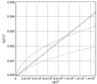Figure 1

The full wave system energy is presented on Figure 1 as the function of spatial coordinate for all three approaches: ZRP, M1 and M2. One can note that ZRP curve reproduces automodel law Eq.(3), corresponding to the index value $p=1$, which is known to correspond to more than a dozen of field experiments, mentioned and processed in [Badulin et al., 2007], and hence should be used as the reference point.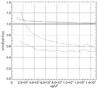Figure 2

The appropriate values of automodel powers $p$ for ZRP, M1 and M2 approaches can be seen on Figure 2. Whereas ZRP approach shows asymptotic merger with automodel theoretical prediction $p=1$, M1 approach fails to do that. M2 approach converges as well, but to another power value $p \simeq 0.5$ with sluggish rate. That examination shows automodel tendencies in ZRP and M2 approaches. One can notice quite good accordance between ZRP and M1 approaches for wave energy behavior on Figure 1 for "experimental" dimensional distances up to $\sim 20$ km, which confirms M1 merits.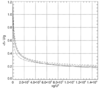Figure 3

Figure 3 presents average frequency dependencies on the coordinate for three considered approaches and demonstrates its $25\%$ scatter for the distances, exceeding $20$ km.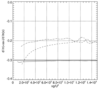Figure 4

Figure 4 shows the dependencies similar to automodel law Eq.(5): ZRP model demonstrates automodel behavior, exhibiting asymptotic evolution to the theoretically predicted power $q = 0.3$, although M1 and M2 approaches have more sluggish evolution to the dissimilar value $q \simeq 0.2$ with better quality of convergence for M2 approach. The undulations observed in the evolution of $q$ on that graph could be connected with discrete wave-numbers, used in the modeling due to sharp spectral peak perpetual shift amid neighboring frequency point, i.e. spectral peak motion in a sort of "hit and miss" manner.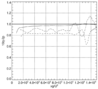Figure 5

The check of calculated "magic number" $(10q-2p)$ (see Eq.(4)) is presented on Figure 5. It exhibits asymptotic convergence of ZRP model to the target value of $1$, while M1 and M2 models converge to the slightly lower values $0.8 \div 0.9$ somewhat slower along the real space coordinate. Particularly obvious in M1 and M2 approaches oscillations can be explained by Fourier space discreteness, discussed above.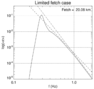Figure 6

Angular averaged wave energy spectrum decimal logarithm for ZRP approach is shown on Figure 6 as the function of frequency decimal logarithm for $x \simeq 20$ km. One can distinguish its following parts:

• {} the spectral peak
• {} the inertial band close to $\omega^{-4}$ stretching from the spectral peak area to the start of the "implicit" wave energy absorption at $f_d = 1.1$ Hz
• {} high-frequency tail $f^{-5}$, beginning from $f_d=1.1$ Hz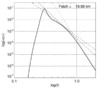Figure 7

Angular averaged wave energy spectrum decimal logarithm for M1 approach is shown on Figure 7 as the dependence on the frequency decimal logarithm, for the coordinate value $x \simeq 20$ km. It can be seen that no any part of the spectral tail can be approximated by $\sim \omega^{-4}$ fitting. The single domain, which could be fitted by power function, is $f>1.1$ Hz, with $\sim \omega^{-8.4}$ spectral dependence. Such rapidly decaying spectra, however, were never seen in the natural experiments.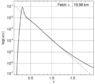Figure 8

Angular averaged wave energy spectrum decimal logarithm, depending on the frequency, for M1 approach, is shown on Figure 8, for the coordinate value $x \simeq 20$ km. The spectral domain $0.5 \,\,\, Hz < f < 1.2 \,\,\, Hz$ can be fitted by $\sim 10^{-3.3 f}$, which has never been seen in the natural experiments either.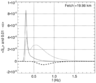Figure 9

Appearance of both exponential and power-like spectra for intermediate and high frequency ranges, correspondingly, for M1 approach, finds its explanation from Figure 9. It shows that significant part of the wave breaking absorption is localized in the area of the intermediate frequencies, right adjacent to the spectral peak area. Such localization of the wave-breaking absorption causes exponential decay at the intermediate frequencies of the spectral tail.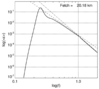Figure 10

Angular averaged wave energy spectrum decimal logarithm, depending on the frequency decimal logarithm, for M2 approach, at the coordinate $x \simeq 20$ km, is shown on Figure 10. As in ZRP approach, one can distinguish the following parts:

• {} the spectral peak
• {} the inertial band close to $\omega^{-4}$, stretching from the spectral peak area to the start of the "implicit" absorption $f_d=1.1$ Hz
• {} high-frequency tail $f^{-5}$, beginning from $f_d=1.1$ Hz

That observation confirms the concept of nonlinear interaction domination in the hierarchy of the source terms in Eq.(1), which exhibits itself in the formation of $\omega^{-4}$ spectral tail in the inertial frequencies range, stretching from the spectral peak area up to the beginning of the absorption range $f_d=1.1$ Hz.

### Conclusions

M. Donelan set of wind input and nonlinear wave-breaking absorption (cited as M1 approach) is one of the well-known and widely cited models, applied to the wave energy transfer equation. We performed numerical comparison of M1 approach with recently developed ZRP approach and "artificial" M2 approach (which is, in fact, the modified M1 approach) for stationary version of energy transfer Eq.(1), describing wind waves excitation off the shore line.

ZRP and M1 approaches use different methods for their derivation: ZRP uses the automodel solutions of HE together with experimental data, while M1 applies purely experimental observations for its composition. The "artificial" M2 approach integrates the properties of ZRP as well as M1 one.

The comparison shows that there is substantial discrepancy between ZRP and M1 approaches. Whereas ZRP model exhibits automodel properties, which are the hallmarks of the nonlinearity, M1 approach demonstrates just a few of them, with slow asymptotic approach to the theoretically predicted values.

In addition, ZRP and M2 exhibit power-like $\omega^{-4}$ spectra, whereas M1 possesses exponentially decaying behavior in the intermediate frequencies range. The reason of exponential tail appearance is dissimilarity in wave energy absorption localization: while ZRP approach relies of high-frequency absorption, M1 model utilizes intermediate wave numbers wave energy absorption. Also, ZRP approach replicates the full wave energy and the average frequency actions of experimentally gathered data.

It is quite interesting, that M2 "artificial" approach enhances automodel properties of M1, i.e. full wave energy, average frequency indices, "magic numbers" as the functions of coordinate automodel behavior, as well as the properties of angular integrated spectra, like $\sim \omega^{-4}$ spectral tails. This observation evidences the fact that the substitution of the quite complex nonlinear wave-breaking absorption in M1 approach by fairly simple "implicit" absorption $\omega^{-5}$ for high wave-numbers spectral tail improves the quality of M1 approach, provided that the wind wave energy input sheltering coefficient is re-tuned.

Outlined research leads to the conjecture that the wide class of source terms, utilized in ensemble with exact expression for nonlinear interaction source term $S_{nl}$ and "implicit" high frequency wave-breaking absorption, results in better consistency with theoretically forecasted automodel properties of the energy transfer equation, spectral shapes and data of the field experiments.

#### Acknowledgments

The authors declare that they have no conflicts of interest. This research was funded by Rissian Science Foundation, grant No 19-72-30028 and MIGO group. The authors gratefully acknowledge the support of these foundations.

### References

Badulin, S., et al. (2007) , Weakly turbulent laws of wind-wave growth, J. Fluid Mech., 591, p. 339–378.

Badulin, S. I., et al. (2005) , Self-similarity of wind-driven sea, Nonlinear Proc. in Geophysics, 12, p. 891–945.

Banner, M. L., R. Young (1975) , Modeling Spectral Dissipation in the Evolution of Wind Waves. Part I: Assessment of Existng Model, JPO, 24, p. 5�6.

Charnock, H. (1955) , Wind stress on a water surface, Q.J.R. Meteorol. Soc., 81, p. 639–640.

Donelan, M. A., et al. (2012) , Modeling waves and wind stress, Journal of Geophysical Research: Oceans, 117, p. C00J23, https://doi.org/10.1029/2011JC007787 (http://dx.doi.org/10.1029/ 2011JC007787).

Dyachenko, A. I., et al. (2015) , Evolution of one-dimensional wind-driven sea spectra, JETP Letters, 102, p. 577–581.

Fedorenko, R. P. (1994) , Introduction into computational physics, Nauka, Moscow (in Russian).

Gagnaire-Renou, E., et al. (2011) , On weakly turbulent scaling of wind sea in simulations of fetch-limited growth, Journal of Fluid Mechanics, 669, p. 178–213, https://doi.org/10.1017/S0022112010004921.

Hasselmann, K. (1962) , On the non-linear energy transfer in a gravity-wave spectrum. Part 1. General theory, Journal of Fluid Mechanics, 12, p. 481–500.

Kats, A. V., V. M. Kontorovich (1974) , Anisotropic turbulent distributions for waves with a non-decay dispersion law, Soviet Physics JETP, 38, p. 102–107.

Kats, A. V., V. M. Kontorovich, et al. (1975) , Power like solutions of the kinetic Boltzmann equation for distributions 20 of particles with spectral fluxes, JETP Letters, 21, p. 5–6.

Phillips, O. M. (1966{}) , The dynamics of the upper ocean, Cambridge monographs on mechanics and applied mathematics, 261 pp., U. P., Oxford.

Pushkarev, A., V. Zakharov (2016) , Limited fetch revisited: comparison of wind input terms, in surface wave modeling, Ocean Modeling, 103, p. 18–37, https://doi.org/10.1016/j.ocemod.2016.03.005.

SWAN: http://swanmodel.sourceforge.net/, 2015.

Tolman H. L. (2013), User manual and system documentation of WAVEWATCH III, Environmental Modeling Center, Marine Modeling and Analysis Branch

Tolman, H. L., D. Chalikov (1996) , Source Terms in a Third-Generation Wind Wave Model, Journal of Physical Oceanography, 26, p. 2497–2518, https://doi.org/10.1175/1520-0485(1996)026%3C2497:STIATG%3E2.0.CO;2.

Tracy, B. and D. Resio (1982), Theory and calculation of the nonlinear energy transfer between sea waves in deep water, WES report 11, U.S. Army Engineer Waterways Experiment Station, Vicksburg, MS/

Zakharov, V., et al. (2017) , Balanced source terms for wave generation within the Hasselmann equation, Nonlin. Processes Geophys., 24, p. 581–597, https://doi.org/10.5194/npg-24-581-2017.

Zakharov, V. E. (2010) , Energy balances in a wind-driven sea, Physica Scripta, T142, p. 014–052.

Zakharov, V. E, S. I. Badulin (2011) , On energy balance in wind-driven sea, Doklady Akademii Nauk, 440, p. 691–695.

Zakharov, V. E., N. N. Filonenko (1967) , The energy spectrum for stochastic oscillations of a fluid surface, Sov. Phys. Docl., 11, p. 881–884.

Received 04 October 2019; accepted 10 October 2019; published 8 December 2019.Citation: Pushkarev Andrei (2019), On different approaches to statistical description of ocean waves, Russ. J. Earth Sci., 19, ES6006, doi:10.2205/2019ES000683.

Generated from LaTeX source by ELXfinal, v.2.0 software package.